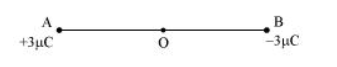# Two point charges

Question:

Two point charges $q_{A}=3 \mu C$ and $q_{B}=-3 \mu C$ are located $20 \mathrm{~cm}$ apart in vacuum.

(a) What is the electric field at the midpoint O of the line AB joining the two charges?

(b) If a negative test charge of magnitude $1.5 \times 10^{-9} \mathrm{C}$ is placed at this point, what is the force experienced by the test charge?

Solution:

(a) The situation is represented in the given figure. O is the mid-point of line AB.Distance between the two charges, AB = 20 cm

$\therefore A O=O B=10 \mathrm{~cm}$

Net electric field at point $O=E$

Electric field at point $O$ caused by $+3 \mu C$ charge,

$E_{1}=\frac{3 \times 10^{-6}}{4 \pi \epsilon_{0}(\mathrm{AO})^{2}}=\frac{3 \times 10^{-6}}{4 \pi \in_{0}\left(10 \times 10^{-2}\right)^{2}} \mathrm{~N} / \mathrm{C}$ along $\mathrm{OB}$

Where,

$\epsilon_{0}=$ Permittivity of free space

$\frac{1}{4 \pi \epsilon_{0}}=9 \times 10^{9} \mathrm{Nm}^{2} \mathrm{C}^{-2}$

Magnitude of electric field at point $O$ caused by $-3 \mu C$ charge,

$E_{2}=\left|\frac{-3 \times 10^{-6}}{4 \pi \in_{0}(\mathrm{OB})^{2}}\right|=\frac{3 \times 10^{-6}}{4 \pi \in_{0}\left(10 \times 10^{-2}\right)^{2}} \mathrm{~N} / \mathrm{C}$ along $\mathrm{OB}$

$\therefore E=E_{1}+E_{2}$

$=2 \times\left[\left(9 \times 10^{9}\right) \times \frac{3 \times 10^{-6}}{\left(10 \times 10^{-2}\right)^{2}}\right]$   [Since the values of $E_{1}$ and $E_{2}$ are same, the value is multiplied with 2]

$=5.4 \times 10^{6} \mathrm{~N} / \mathrm{C}$ along $\mathrm{OB}$

Therefore, the electric field at mid-point $O$ is $5.4 \times 10^{6} \mathrm{~N} \mathrm{C}^{-1}$ along $\mathrm{OB}$.

(b) A test charge of amount $1.5 \times 10^{-9} \mathrm{C}$ is placed at mid-point $\mathrm{O}$.

$q=1.5 \times 10^{-9} \mathrm{C}$

Force experienced by the test charge $=F$

$\therefore F=q E$

$=1.5 \times 10^{-9} \times 5.4 \times 10^{6}$

$=8.1 \times 10^{-3} \mathrm{~N}$

The force is directed along line OA. This is because the negative test charge is repelled by the charge placed at point B but attracted towards point A.

Therefore, the force experienced by the test charge is $8.1 \times 10^{-3} \mathrm{~N}$ along $\mathrm{OA}$.